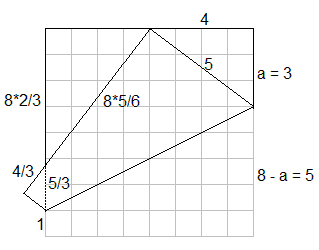# Egyptian Triangle By Paper Folding III

What is known as Haga's First Theorem is a two fold construction of the 3-4-5 ("Egyptian") triangle from a square piece of paper. The construction I believe beats hands down tying knots on a rope (a procedure assumed to be used by the ancient Egyptian and which gave them the moniker of "rope-stretchers".)

The first fold in Haga's construction is to mark the midpoint of a side (the top side in the diagram below). The second fold places one of the lower corners at the midpoint of the top edge:This simple procedure generates a 3-4-5 triangle (three of them naturally, as there are similar triangles.)Assume the side of the stare is $8$ units of length. Let the vertical side of the right triangle in the top right corner be $a.$ Then its hypotenuse equals $8-a$ (which is the remaining part of the vertical side of the square.) The Pythagorean theorem then gives an equation

$a^{2}+4^{2}=(8-a)^2.$

This simplifies to $16=64-16a,$ from which $a=3,$ as shown in the diagram.

[Haga, p.6] draws attention to the fact that the same folding procedure reveals several fractions, e.g. $2/3$ and $1/8.$ The triangle in the upper left corner has the same proportions: $3:4:5$ but its smallest side is now $4,$ implying that the vertical leg equals $4\cdot 4/3=8\cdot 2/3$ so that the vertical leg is $2/3$ of the side of the square. The hypotenuse of that triangle equals $4\cdot 5/3=8\cdot 5/6,$ i.e. $5/6$ of the side of the square. Thus the long leg of the small protruding triangle at the left edge of the square is $1/6$ of the side of the square, making the short side $1/8$ and the hypotenuse $5/24.$ It follows that the second fold marks exactly $8\cdot 1/8=1$ unit up from the bottom edge of the square.

### References

1. Kazuo Haga. et al, Origamics: Mathematical Explorations Through Paper Folding, World Scientific Publishing Company, 2008Paper Folding Geometry